• ## 递归算法时间复杂度分析

万次阅读 多人点赞 2018-09-17 16:16:59
递归算法时间复杂度分析 时间复杂度： 一般情况下，算法中基本操作重复的次数就是问题规模n的某个函数f（n），进而分析f（n）随n的变化情况并确定T（n）的数量级。这里用‘o’来表示数量级，给出算法时间复杂度。 T...
                             递归算法时间复杂度分析

时间复杂度：

一般情况下，算法中基本操作重复的次数就是问题规模n的某个函数f（n），进而分析f（n）随n的变化情况并确定T（n）的数量级。这里用‘o’来表示数量级，给出算法时间复杂度。
T（n）=o（f（n））；
它表示随问题规模n的增大，算法的执行时间增长率和f（n）增长率成正比，这称作算法的渐进时间复杂度。而我们一般情况下讨论的最坏的时间复杂度。
空间复杂度：
算法的空间复杂度并不是实际占用的空间，而是计算整个算法空间辅助空间单元的个数，与问题的规模没有关系。算法的空间复杂度S（n）定义为该算法所耗费空间的数量级。
S（n）=o（f（n））
若算法执行所需要的辅助空间相对于输入数据n而言是一个常数，则称这个算法空间复杂度辅助空间为o（1）；
递归算法空间复杂度：递归深度n*每次递归所要的辅助空间，如果每次递归所需要的辅助空间为常数，则递归空间复杂度o（n）。

递归算法分析

1、利用数列知识

累加法：递推关系式为an+1−an=f(n)an+1−an=f(n)采用累加法。
累乘法：递推关系式为an+1an=f(n)an+1an=f(n)采用累乘法。
构造法：递推关系式为(1)aa+1=pan+qaa+1=pan+q，(2)aa+1=pan+qnaa+1=pan+qn，都可以通过恒等变形，构造出等差或等比数列，利用等差或等比数列的定义进行解题，其中的构造方法可通过待定系数法来进行。
和化项法：递推公式为Sn=f(n)Sn=f(n)或Sn=f(an)Sn=f(an)一般利用
an={S1，Sn−Sn−1，当n=1当n>=2an={S1，当n=1Sn−Sn−1，当n>=2

用特征方程求解递推方程（感觉比较生僻，不做解释）
迭代法： 从原始递推方程开始，反复将对于递推方程左边的函数用右边的等式代入，直到得到初值，然后将所得的结果进行化简。
例如在调用归并排序mergeSort(a,0,n-1)对数组a[0...n−1]a[0...n−1]排序时，执行时间T(n)T(n)的递推关系式为：
T(n)={O(1)，2T(n2)+O(n)，当n=1当n>=2T(n)={O(1)，当n=12T(n2)+O(n)，当n>=2

其中，O(n)O(n)为merge()所需要的时间，设为cncn（c为正常量）。因此：
T(n)=2T(n2)+cn=2(2T(n4)+cn2)+cn=22T(n4)+2cn=23T(n8)+3cn=...=2kT(n2k)+kcn=nO(1)+cnlog2n=O(nlog2n),(假设n=2k,则k=log2n)T(n)=2T(n2)+cn=2(2T(n4)+cn2)+cn=22T(n4)+2cn=23T(n8)+3cn=...=2kT(n2k)+kcn=nO(1)+cnlog2⁡n=O(nlog2⁡n),(假设n=2k,则k=log2⁡n)

忽略求解细节。在我们求解递归式时，因为最终是要求得一个时间上限，所以在求解时常常省略一些细节。比如mergeSort(a,0,n-1)运行时间的实际递归式应该是：

T(n)={O(1)，T(⌈n2⌉)+T(⌊n2⌋)+O(n)，当n=1当n>=2T(n)={O(1)，当n=1T(⌈n2⌉)+T(⌊n2⌋)+O(n)，当n>=2

但我们忽略这些上取整、下取整以及边界条件，甚至假设问题规模n=2kn=2k，这都是为方便求解而忽略的细节。经验和一些定理告诉我们，这些细节不会影响算法时间复杂度的渐近界。

类似的，我们也可以用迭代法求解汉诺塔递归求解时的时间复杂度。但遗憾的是，迭代法一般适用于一阶的递推方程。对于二阶及以上（即T(n)依赖它前面更多个递归项T(n)依赖它前面更多个递归项）的递推方程，迭代法将导致迭代后的项太多，从而使得求和公式过于复杂，因此需要将递推方程化简，利用差消法等技巧将高阶递推方程化为一阶递推方程。如在求快速排序算法平均时间复杂度T(n)T(n)的递推方程，T(n)T(n)依赖T(n−1)、T(n−2)、...、T(1)T(n−1)、T(n−2)、...、T(1)等所有的项，这样的递推方程也称为全部历史递推方程。（这里省略快速排序算法平均复杂度T(n)的求解过程）

小结：上面6种递推关系是高中、本科知识，在此重点介绍了迭代法，其它几种方法虽未在本篇中使用，但可以加深对递推式求解的认识。

2、代入法

代入法实质上就是数学归纳法，因此求递推式分为两步：

猜测解的形式；
用数学归纳法求出解中的常数，并证明解是正确的。
遗憾的是并不存在通用的方法来猜测递归式的正确解，需要凭借经验，偶尔还需要创造力。即使猜出了递归式解的渐近界，也有可能在数学归纳证明时莫名其妙的失败。正是由于该方法技术细节较为难掌握，因此这个方法不适合用来求解递归方程，反而比较适合作为其他方法检验手段。在此不做总结。可以翻阅《算法导论》进行学习。

3、递归树

递归树是一棵结点带权值的树。初始的递归树只有一个结点，它的权标记为T(n)T(n)；然后按照递归树的迭代规则不断进行迭代，每迭代一次递归树就增加一层，直到树中不再含有权值为函数的结点（即叶结点都为T(1)T(1)）。下面以递归方程

T(n)={O(1)，2T(n2)+O(n)，当n=1当n>=2;(假设n=2k,则k=log2n)T(n)={O(1)，当n=12T(n2)+O(n)，当n>=2;(假设n=2k,则k=log2⁡n)

来讲述递归树的迭代规则。

第一步： 把根结点T(n)T(n)用根是cncn、左结点为T(n2)T(n2)、右结点为T(n2)T(n2)的子树代替（即：以分解、合并子问题需要的代价为根，分解得到的子问题为叶的子树。其中常量c代表求解规模为1的问题所需的时间）；（如下如(a)→(b)(a)→(b)）
第二步：把叶结点按照“第一步”的方式展开；T(n2)T(n2)用根是cn/2cn/2、左节点为T(n4)T(n4)、右结点为T(n4)T(n4)的子树代替。（如下如(b)→(c)(b)→(c)）
第三步：反复按照“第一步”的方式迭代，每迭代一次递归树就增加一层，直到树中不再含有权值为函数的结点（即叶结点都为T(1)T(1)）。（如下如(c)→(d)(c)→(d)）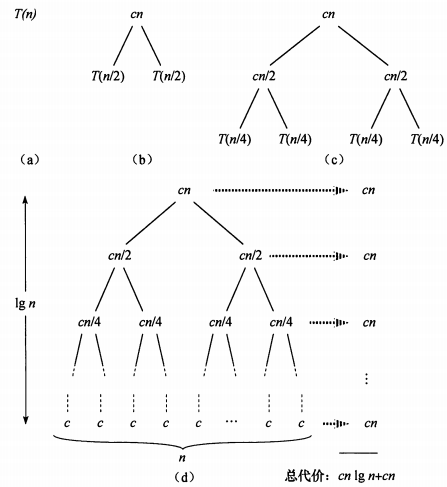在得到递归树后，将树中每层中的代价求和，得到每层代价，然后将所有层的代价求和，得到所有层次的递归调用的总代价。在上图(d)部分中，完全展开的递归树高度为lgnlg⁡n(树高为根结点到叶结点最长简单路径上边的数目)，所有递归树具有lgn+1lg⁡n+1层，所以总代价为cn∗(lgn+1)cn∗(lg⁡n+1)，所有时间复杂度为Θ(nlgn)Θ(nlg⁡n)。

总结：递归树模型求解递归方程，本质上就是迭代思想的应用，利用递归方程迭代展开过程构造对应的递归树，然后把每层的时间代价进行求和。不过递归树模型更直观，同时递归树也克服了二阶及更高阶递推方程不方便迭代展开的痛点。

4、主方法求解递推式

主方法为如下形式的递归式提供了一种“菜谱”式的求解方法，如下所示

T(n)=aT(n/b)+f(n)T(n)=aT(n/b)+f(n)

其中a≥1a≥1和b>1b>1是常数，f(n)f(n)是渐近正函数。这个递推式将规模为n的问题分解为a个子问题，每个子问题的规模为n/bn/b，a个子问题递归地求解，每个花费时间T(n/b)T(n/b)。函数f(n)f(n)包含了问题分解和子问题解合并的代价。同样，这个递归式也没有考虑上取整、下取整、边界条件等，结果不会影响递归式的渐近性质。

定理4.1(主定理) 令a≥1和b>1是常数，f(n)f(n)是一个函数，T(n)T(n)是定义在非负整数上的递归式：

T(n)=aT(n/b)+f(n)T(n)=aT(n/b)+f(n)

其中我们将n/bn/b解释为⌊n/b⌋⌊n/b⌋或⌈n/b⌉⌈n/b⌉。那么T(n)T(n)有如下渐近界：
1. 若对某个常数ε>0ε>0有f(n)=O(n(logba)−ε)f(n)=O(n(logb⁡a)−ε)，则T(n)=Θ(nlogba)T(n)=Θ(nlogb⁡a)
2. 若f(n)=Θ(nlogba)f(n)=Θ(nlogb⁡a)，则T(n)=Θ(nlogbalgn)T(n)=Θ(nlogb⁡alg⁡n)。
3. 若对某个常数ε>0ε>0有f(n)=Ω(n(logba)+ε)f(n)=Ω(n(logb⁡a)+ε)，且对某个常数c<1c<1和所有足够大的n有af(n/b)≤cf(n)af(n/b)≤cf(n)，则T(n)=Θ(f(n))T(n)=Θ(f(n))

在使用主定理之前，要比较f(n)和(nlogba)f(n)和(nlogb⁡a)的大小，这个大小不是算术意义上的大小比较，而是要在多项式意义上比较。以上三种情况在多项式意义上并未覆盖f(n)f(n)的所有可能性。情况1和情况2之间有一定间隙；情况2和情况3之间也有一定间隙。如果f(n)落在这两个间隙中，或者情况3中 正则条件不成立，就不能使用主方法来求递归式。
如在递归式：T(n)=2T(n/2)+nlgnT(n)=2T(n/2)+nlg⁡n中，因为 nlogba=n<f(n)=nlgnnlogb⁡a=n<f(n)=nlg⁡n，但是f(n)f(n)并不大于n一个多项式因子nεnε ,因为对于给定的ε>0ε>0当n足够大时，均有nε>lgnnε>lg⁡n。所以找不到这样 ε>0ε>0，该递归式落入了情况2和情况3之间的间隙，不能使用主定理。
最后给出主定理应用的几个练习题：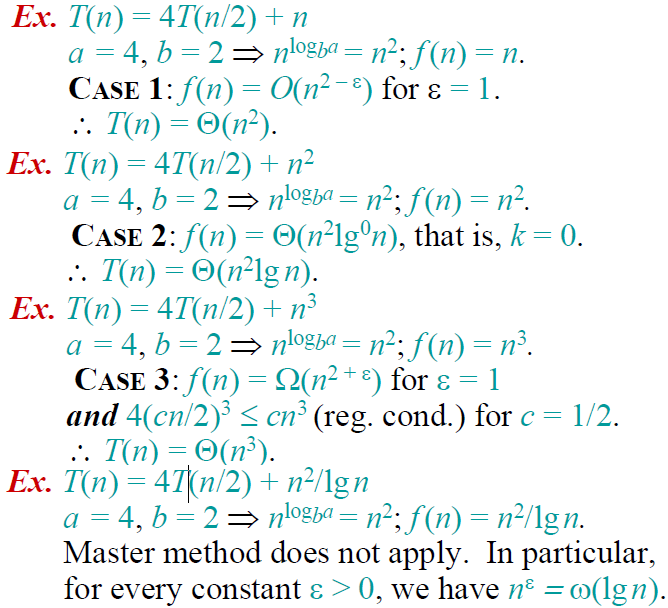具体举例分析：

【代入法】代入法首先要对这个问题的时间复杂度做出预测，然后将预测带入原来的递归方程，如果没有出现矛盾，则是可能的解，最后用数学归纳法证明。

【举   例】我们有如下的递归问题：T(n)=4T(n/2)+O(n)，我们首先预测时间复杂度为O(n2),不妨设T(n)=kn2（其中k为常数），将该结果带入方程中可得：左=kn2，右=4k(n/2)2+O(n)=kn2+O(n),由于n2的阶高于n的阶，因而左右两边是相等的，接下来用数学归纳法进行验证即可。

【迭代法】迭代法就是迭代的展开方程的右边，直到没有可以迭代的项为止，这时通过对右边的和进行估算来估计方程的解。比较适用于分治问题的求解，为方便讨论起见，给出其递归方程的一般形式：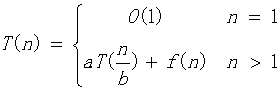【举   例】下面我们以一个简单的例子来说明：T(n)=2T(n/2)+n2,迭代过程如下：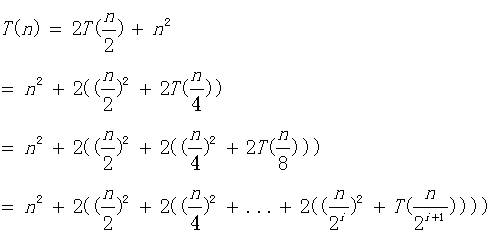容易知道，直到n/2^(i+1)=1时，递归过程结束，这时我们计算如下：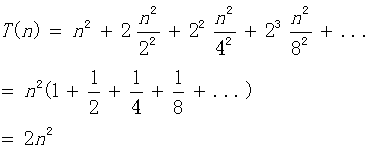到这里我们知道该算法的时间复杂度为O(n2)，上面的计算中，我们可以直接使用无穷等比数列的公式，不用考虑项数i的约束，实际上这两种方法计算的结果是完全等价的，有兴趣的同学可以自行验证。

【公式法】这个方法针对形如：T(n) = aT(n/b) + f(n)的递归方程。这种递归方程是分治法的时间复杂性所满足的递归关系，即一个规模为n的问题被分成规模均为n/b的a个子问题，递归地求解这a个子问题，然后通过对这a个子问题的解的综合，得到原问题的解。这种方法是对于分治问题最好的解法，我们先给出如下的公式：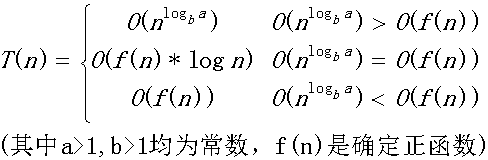公式记忆：我们实际上是比较n^logba和f(n)的阶，如果他们不等，那么T(n)取他们中的较大者，如果他们的阶相等，那么我们就将他们的任意一个乘以logn就可以了。按照这个公式，我们可以计算【迭代法】中提到的例子：O(f(n))=O(n2),容易计算另外一个的阶是O(n),他们不等，所以取较大的阶O(n2)。太简单了，不是吗？

需要注意：上面的公式并不包含所有的情况，比如第一种和第二种情况之间并不包含下面这种情况：f(n)是小于前者，但是并不是多项式的小于前者。同样后两种的情况也并不包含所有的情况。为了好理解与运用的情况下，笔者将公式表述成如上的情况，但是并不是很严谨，关于该公式的严密讨论，请看这里。但是公式的不包含的情况，我们很少很少碰到，上面的公式适用范围很广泛的。

特别地，对于我们经常碰到的，当f(n)=0时，我们有：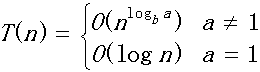【母函数法】母函数是用于对应于一个无穷序列的幂级数。这里我们解决的递归问题是形如：T(n)=c1T(n-1)+c2T(n-2)+c3T(n-3)+...+ckT(n-k)+f(n)。为说明问题简便起见，我们选择斐波那契数列的时间复杂度作为例子进行讨论。

【举  例】斐波那契数列递归公式：T(n)=T(n-1)+T(n-2)。这里我们假设F(n)为第n项的运算量。则容易得到：F(n)=F(n-1)+F(n-2),其中F(1)=F(2)=1.我们构造如下的母函数：G(x)=F(1)x+F(2)x2+F(3)x3+......,我们可以推导如下：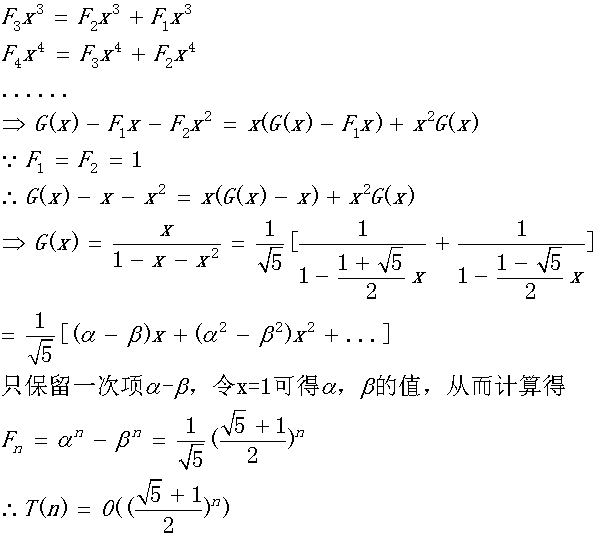上面的方法计算相对来说是比较简单的，关键在于对于母函数的理解，刚开始的时候可能不是很好理解，对于母函数可以参考这里和维基百科这里。

【差分方程法】可以将某些递归方程看成差分方程，通过解差分方程的方法来解递归方程，然后对解作出渐近阶估计。这里我们只考虑最长常见的递归形式，形如：T(n)=c1T(n-1)+c2T(n-2)+c3T(n-3)+...+ckT(n-k)+f(n)，其中c1,c2,...ck为常数且不等于0；我们对该方程的求解如下：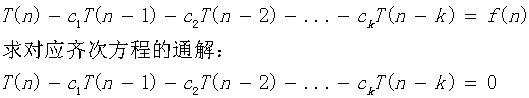对应上面的齐次方程的特征方程为：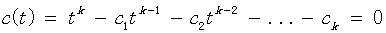如果解得t=r是该特征方程的m重根，则这m个解的形式为：{rn     n*rn      n2rn   ...    nm-1rn},其余的关于复数解的形式和普通的线性方程组的形式类似，不再赘述。接下来，我们要求解该方程的对应非齐次方程组的通解，这里我们针对该方程的特殊形式，不加证明地给出如下的通解形式：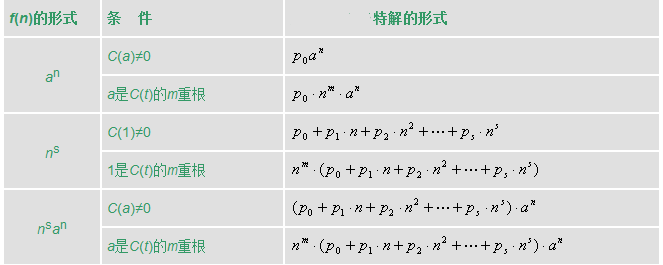则和线性代数中的解一样，原方程的解等于齐次方程组的通解+特解，即：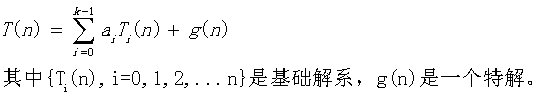最后由初始条件确定a(i)的值即可。

为了帮助理解，我们举两个例子看看，就明白是怎么回事了。

【举 例1】递归方程如下：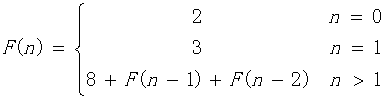(1)写出对应齐次方程的特征方程：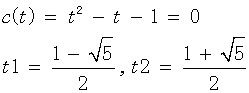得到基础解系为：{t1n,  t2n}

(2)计算特解，对于本题，直接观察得特解为：-8

(3)得到原方程解的形式为：T(n)=a0t1n+a1t2n-8

(4)代入n=0，n=1的情况，得到a0,a1，最后可得: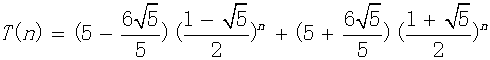可以看到该方程形式和上面讨论过的斐波那契数列只差一个常数8，因而两者的时间复杂度是相同的。有兴趣的同学可以按照这个方法再次计算斐波那契数列的时间复杂度验证一下。

【举  例2】递归方程如下：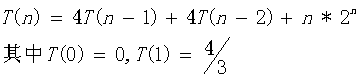（1）计算对应齐次方程的基础解析：特征方程为：C(t)=t^2-4t-4=0,得到一个2重根t=2.因而其基础解为：{2n      n*2n}

（2）由于f(n)=n*2n，对应上面表格的最后一种情况，得到特解形式为：T(n)=n2(p0+p1n)2n代入原递归方程可得：p0=1/2,p1=1/6

（3）原方程解的形式为：T(n)=a0*2n+a1*n*2n+n2(1/2+n/6)2n,代入T(0),T(1)得：a0=a1=0

（4）综上：T(n)=n2(1/2+n/6)2n

因而时间复杂度为：O(n32n).

参考：https://blog.csdn.net/so_geili/article/details/53444816

https://blog.csdn.net/m0_37734999/article/details/78751882

https://www.cnblogs.com/hlongch/p/5749038.html

展开全文• 递归实现递归二分查找： 时间复杂度为O(logn) 时间复杂度为O(1) 2、斐波那契数列 递归 时间复杂度为O(2^n) 时间复杂度为O(1) 非递归 时间复杂度为O(n) 时间复杂度为O(1) 总结1、时间复杂度就是一...
1、二分查找

非递归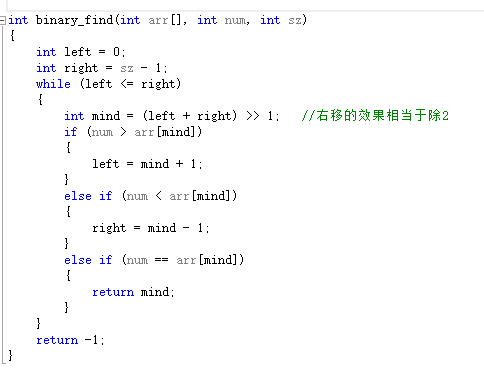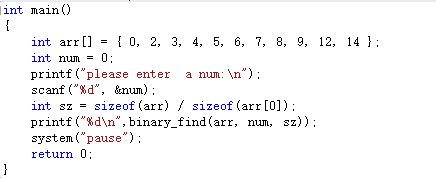非递归二分查找：
时间复杂度为O(logn)
空间复杂度为O(1)

递归实现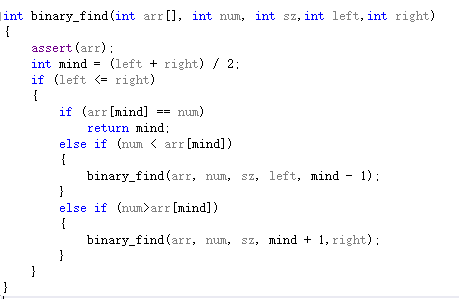非递归二分查找：
时间复杂度为O(logn)
空间复杂度为O(1)
2、斐波那契数列

递归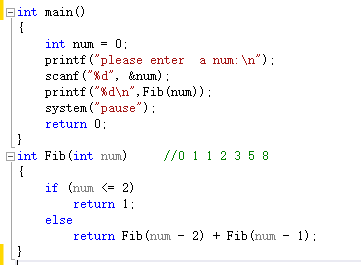时间复杂度为O(2^n)
空间复杂度为O(1)

非递归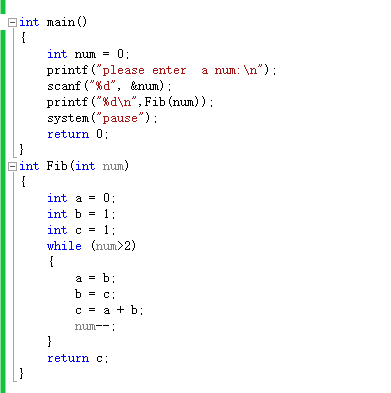时间复杂度为O(n)
空间复杂度为O(1)
总结

1、时间复杂度就是一个计算执行基本操作的次数的函数 一般算法O(n)计算方法：
<1>用时间1取代运行时间中的所有加法常数

<2>在修改后的运行次数函数中，只保留最高阶项

例如：2*n^3+n （只保留n^3,因为n^3的增长率远远大于n）

<3>如果最高阶项系数存在且不是1，则去除与这个项相乘的常数
(所以上式的2可以除去，时间复杂度为0(n^3) )

2、递归算法时间复杂度=递归总次数*每次递归次数

3、空间复杂度：函数中创建对象的个数关于问题规模函数表达式
展开全文二分查找
• JS递归代码实现 当参数为n时，时间复杂度为f(n) = f(n-1) + f(n-2) 当n为6时，树的高度为5即h=n-1的高度，共有15个节点即2^(h-1)-1个 时间复杂度为O(2^n) = f(2^n-1) - 1 空间复杂度为O(n) = f(n-1)
JS递归代码实现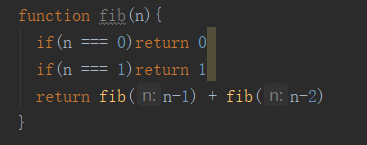当参数为n时，时间复杂度为f(n) = f(n-1) + f(n-2)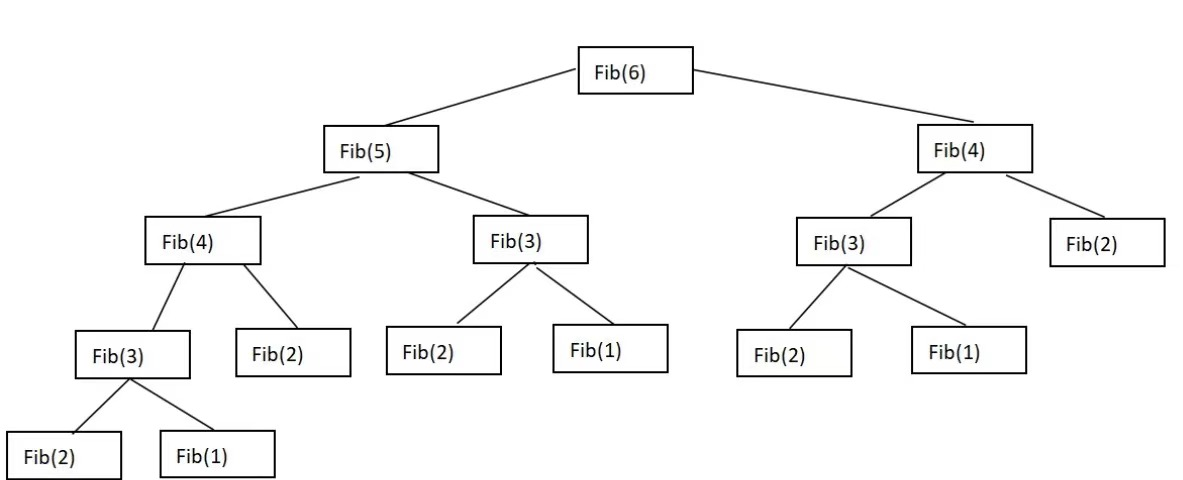当n为6时，树的高度为5即h=n-1的高度，共有15个节点即2^(h-1)-1个

时间复杂度为O(2^n) = f(2^n-1) - 1

空间复杂度为O(n) = f(n-1)

展开全文数据结构 算法 javascript
• 斐波那契数列——递归时间复杂度计算 画图：以F（6）为例：斐波那契数列到第六个数停止 每个节点运行都会开辟空间，使用时间 为了方便计算，把第五层的f（2）和f（1）放在第四层最右边，不会影响时间复杂度计算 ...
斐波那契数列——递归法时间复杂度计算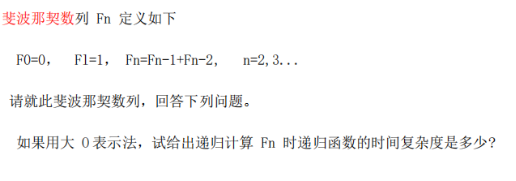画图：以F（6）为例：斐波那契数列到第六个数停止
每个节点运行都会开辟空间，使用时间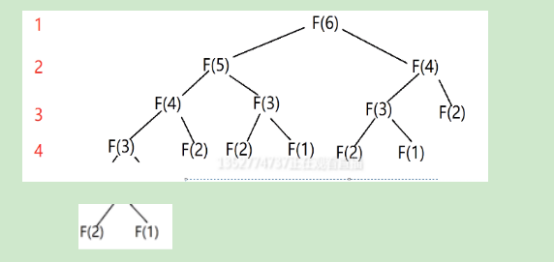为了方便计算，把第五层的f（2）和f（1）放在第四层最右边，不会影响时间复杂度计算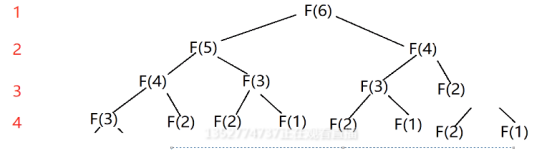第n层节点个数：2^n 个
前n层节点个数：1+2+4+……+2^n  =  (2 ^ n) - 1
F(6)有4层，推理：F（n） 有 n-2层
则F（n）一共有节点数  (2 ^ （n-2）) - 1 =  ((2 ^n)/4)-1
计算时间复杂度规则：不看常数，不看系数，只看最高次数项
因此：O(F(n)) =  O(2 ^n)


展开全文• int f(unsigned int n) { ++c[n]; if(n <= 1) { return 1; } return f(n-1) + f(n-2); }shell
• ## 用递归树求解递归算法时间复杂度

千次阅读 多人点赞 2019-07-01 23:27:38
递归代码复杂度分析起来比较麻烦。一般来说有两种分析方法：递推公式和递归树。 1 递推公式法 归并排序的递推公式是： merge_sort(p…r) = merge(merge_sort(p…q), merge_sort(q+1…r)) 终止条件： p >= r ...
• 发现我们对递归时间复杂度比较陌生，不知道如何分析。 先看看常见的几个例子： 1、从1加到100 public static int function(int n) { //递归结束条件 .即 fun(0) = 0 if (n == 0) return 0; return function(n...
• 斐波那契数列是这样一个数列：1,1,2,3,5,8,13,21,34,55,89,144,233,377,610,987,1597,2584,4181,6765… 表达式为： (注：斐波那契数列也可从n=0开始，对应的F(0)=0) ...//递归实现斐波那契数列 int fib1(...数据结构 算法
• 递归算法时间复杂度的计算方程式一个递归方程： 　 　在引入递归树之前可以考虑一个例子： 　T(n) = 2T(n/2) + n2 　迭代2次可以得： 　T(n) = n2 + 2(2T(n/4) + (n/2) 2) 　还可以继续迭代，将其完全展开...
• 一、递归 概念：函数自身调用自身 二、时间复杂度 概念：执行的次数和问题规模之间的函数关系，它定量描述了该算法的运行时间。  （1）只考虑高阶项，低阶项直接丢弃；   （2）不要系数 三、空间...
• 在这个过程中，频繁地碰到到递归思想和分治思想，惊讶于这两种的思想的伟大与奇妙的同时，经常要面对的一个问题就是，对于一个给定的递归算法或者用分治思想缩小问题规模的算法，如何求解这个算法的时间复杂度呢？...迭代 C
• 又因为递归实现起来代码比较简洁，所以通常都会使用递归来解决上述问题。比如斐波那契数列，再比如树的前序、中序、后续遍历算法。 递归算法虽然是有代码简洁这个优点，但是其缺点显著。因为递归函数是在执行过程...
• 给出一个递归算法，其时间复杂度 O(T) 通常是递归调用的数量（记作 R） 和计算的时间复杂度的乘积（表示为 O(s)）的乘积： O(T)=R∗O(s) 示例 也许你还记得，在反转字符串问题中，我们需要以相反的顺序打印字符串，...
• 这是我见过对递归算法和时空复杂度介绍的很好的一个文章。 -------------------------------------------------------------------------------------- ...斐波那契时间复杂度和空间复杂度分析 我的笔记： Java.
• 递归算法的时间复杂度除非只有前两项，否则都不是线性的，并且相当耗费内存。我们用最常见的的fibonacci数列来说明： function fibonacci(n){ if( n === 0 || n === 1){ return n; } else { return ...c语言
• 斐波拉契数列的实现时间复杂度的分析算是一个难点，在考研数据结构中也经常会碰到，今天我们就来仔细分析下和解决掉这个问题。 1. 首先，我们先来看看递归形式斐波拉契数列的C语言实现： # include<stdio.h>...c语言 数据结构 算法
• 试分别分析两种算法的时间复杂度。   递归方式 递归方式代码： 递归结束条件可以不同，如果数列从第一个开始且为1，那么就是如下结束条件。 如果从第0个开始且第0个为0，那么结束条件就会改变：n等于0时返回0...
• 斐波那契数列：前两项是1，后面的每项是其前两项之和。比如：1 1 2 3 5 8 13… 递归实现： def Fab(n): ...递归算法时间复杂度为(二叉树的节点个数）：O()=(2h)-1=2n。空间复杂度为树的高度：h即o(n). ...
• 问了个面过boss面的版友，没回我，我还是挺想知道我做的对不对的。 最后一道题，是不是a=0，就是a-1？a1-a2我loop（a2) a1=0;...对了，还有一个求算法复杂度的问题，类似于递归Fibonacci函数的那种return
• #include int fib( int n){ if (n< 2 ) return n; else return fib(n- 1 ) + fib(n- ...时间复杂度为 O(2ⁿ); 转载于:https://www.cnblogs.com/zychengzhiit1/p/5772615.html...